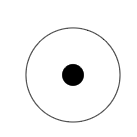# RRB Group-D 3 Oct 2018-shift -2

Instructions

For the following questions answer them individually

Question 21

If $$G$$ is the G.M. of the product of $$r$$ sets of observations with geometric means $$G_1,G_2,G_3,.....G_r$$ respectively, then find the value of $$G$$?

Question 22

The given symbol represents ............ element.Question 23

Name the author of the book 'One Indian Girl'.

Question 24

The recipients of the Whitley Awards, also called the Green Oscar Awards, are:

Question 25

In the Modem Periodic Table, which group has a completely filled valence shell and chemically inactive elements?

Question 26

The speed of a boat in still water is 12 km/h. If the boat covers a distance of 38 km upstream in 4 hours; then the speed of the stream (in km/h) is:

Question 27

When light travels from a rarer medium to a denser medium, it .............

Question 28

Which of the following sports is Gurmeet Singh associated with?

Question 29

Solve the following equation to find x:
$$(x — 2)^2 — 36 = 0$$; x € N

Question 30

What type of tissue are our bones?

OR